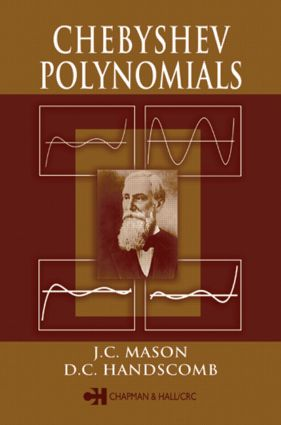# Chebyshev Polynomials

## 1st Edition

Chapman and Hall/CRC

360 pages | 27 B/W Illus.

Hardback: 9780849303555
pub: 2002-09-17
SAVE ~\$37.00
\$185.00
\$148.00
x
eBook (VitalSource) : 9780429191381
pub: 2002-09-17
from \$28.98

FREE Standard Shipping!

### Description

Chebyshev polynomials crop up in virtually every area of numerical analysis, and they hold particular importance in recent advances in subjects such as orthogonal polynomials, polynomial approximation, numerical integration, and spectral methods. Yet no book dedicated to Chebyshev polynomials has been published since 1990, and even that work focused primarily on the theoretical aspects. A broad, up-to-date treatment is long overdue.

Providing highly readable exposition on the subject's state of the art, Chebyshev Polynomials is just such a treatment. It includes rigorous yet down-to-earth coverage of the theory along with an in-depth look at the properties of all four kinds of Chebyshev polynomials-properties that lead to a range of results in areas such as approximation, series expansions, interpolation, quadrature, and integral equations. Problems in each chapter, ranging in difficulty from elementary to quite advanced, reinforce the concepts and methods presented.

Far from being an esoteric subject, Chebyshev polynomials lead one on a journey through all areas of numerical analysis. This book is the ideal vehicle with which to begin this journey and one that will also serve as a standard reference for many years to come.

### Reviews

"The book presents a wide panorama of the applications of Chebyshev polynomials to scientific computing. [It] is very clearly written and is a pleasure to read. Examples inserted in the text allow one to test his or her ability to understand and use the methods, which are described in detail, and each chapter ends with a section full of very pedagogical problems."

Mathematics of Computation

"The book, by two well known specialists, is well written and presented. Many examples are given and problems have been added for students. It is a book that every numerical analyst should have."

- Numerical Algorithms

"… a modern treatment of the subject … in a carefully prepared way … very well produced … "

- Mathematical Reviews, Issue 2004h

DEFINITIONS

Preliminary Remarks

Trigonometric Definitions and Recurrences

Shifted Chebyshev Polynomials

Chebyshev Polynomials of a Complex Variable

BASIC PROPERTIES AND FORMULAE

Introduction

Chebyshev Polynomial Zeros and Extrema

Chebyshev Polynomials and Power of x

Evaluation of Chebyshev Sums, Products, Integrals and Derivatives

MINIMAX PROPERTIES AND ITS APPLICATIONS

Approximation-Theory and Structure

Best and Minimax Approximation

The Minimax Property of the Chebyshev Polynomials

The Chebyshev Semi-Iterative Method for Linear Equations

Telescoping Procedures for Power Series

The Tau Method for Series and Rational Functions

ORTHOGONALITY AND LEAST-SQUARES APPROXIMATION

Introduction-From Minimax to Least Squares

Orthogonality of Chebyshev Polynomials

Orthogonal Polynomials and Best L2 Approximations

Recurrence Relations

Rodriques' Formulae and Differential Equations

Discrete Orthogonality of Chebyshev Polynomials

Discrete Chebyshev Transforms and the FFT

Discrete Data Fitting by Orthogonal Polynomials-The Forsythe-Clenshaw Methods

Orthogonality in the Complex Plane

CHEBYSHEV SERIES

Introduction-Chebyshev Series and Other Expansions

Some Explicit Chebyshev Series Expansions

Fourier-Chebyshev Series and Fourier Theory

Projections and Near-Best Approximations

Near-Minimax Approximation by a Chebyshev Series

Comparison of Chebyshev and Other Polynomial Expansions

The Error of a Truncated Chebyshev Expansion

Series of Second-, Third-, and Fourth-Kind Polynomials

Lacunary Chebyshev Series

Chebyshev Series in the Complex Domain

CHEBYSHEV INTERPOLATION

Polynomial Interpolation

Orthogonal Interpolation

Chebyshev Interpolation Formulae

Best L1 Approximation by Chebyshev Interpolation

Near-Minimax Approximation by Chebyshev Interpolation

NEAR-BEST L8, L1, and Lp APPROXIMATIONS

Near-Best L8 (Near-Minimax) Approximations

Near-Best L1 Approximations

Best and Near-Best Lp Approximations

INTEGRATION USING CHEBYSHEV POLYNOMIALS

Indefinite Integration with Chebyshev Series

Error Estimation for Clenshaw-Curtis Methods

Some other Work on Clenshaw-Curtis Methods

SOLUTION OF INTEGRAL EQUATIONS

Introduction

Fredholm Equations of the Second Kind

Fredholm Equations of the Third Kind

Fredholm Equations of the First Kind

Singular Kernels

Regularisation of Integral Equations

Partial Differential Equations and Boundary Integral Equation Methods

SOLUTION OF ORDINARY DIFFERENTIAL EQUATIONS

Introduction

A Simple Example

The Original Lanczos Tau Method

A More General Linear Equation

Pseudospectral Methods-Another Form of Collocation

Nonlinear Equations

Eigenvalue Problems

Differential Equations in One Space and One Time Dimension

CHEBYSHEV AND SPECTRAL METHODS FOR PARTIAL DIFFERENTIAL EQUATIONS

Introduction

Interior, Boundary, and Mixed Methods

Differentiation Matrices and Nodal Representation

Method of Weighted Residuals

Chebyshev Series and Galerkin Methods

Collocation/Interpolation and Related Methods

PDE Methods

Some PDE Problems and Various Methods

Computational Fluid Dynamics

Particular Issues in Spectral Methods

CONCLUSION

BIBLIOGRAPHY

APPENDICES

Biographical Note

Summary of Notations, Definitions, and Important Properties

Tables of Coefficients

INDEX

Each chapter also contains a section of Problems.